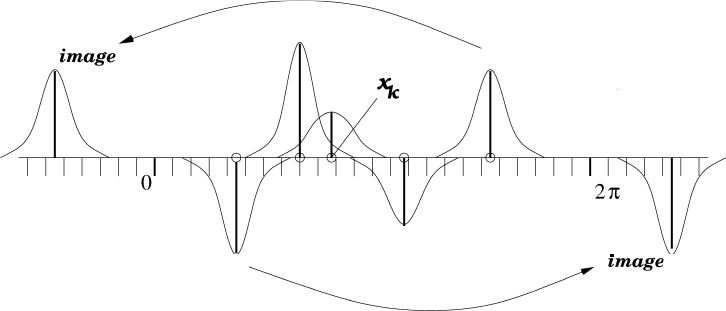## NUFFT (NFFT, USFFT) Software

Fourier analysis plays a natural role in a wide variety of applications, from medical imaging to radio astronomy, data analysis and the numerical solution of partial differential equations. When the sampling is uniform and the Fourier transform is desired at equispaced frequencies, the classical fast Fourier transform (FFT) has played a fundamental role in computation.

The FFT requires O(N log N) work to compute N Fourier modes from N data points rather than O(N2) work.

When the data is irregular in either the "physical" or "frequency" domain, unfortunately, the FFT does not apply. Over the last twenty years, a number of algorithms have been developed to overcome this limitation - generally referred to as non-uniform FFTs (NUFFT), non-equispaced FFTs (NFFT) or unequally-spaced FFTs (USFFT). They achieve the same O(N log N) computational complexity, but with a larger, precision-dependent, and dimension-dependent constant.

We have developed some NUFFT libraries in Fortran 77 and Fortran 90 that are freely available under the GPL license.